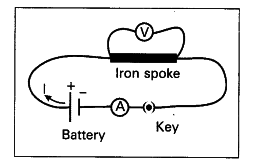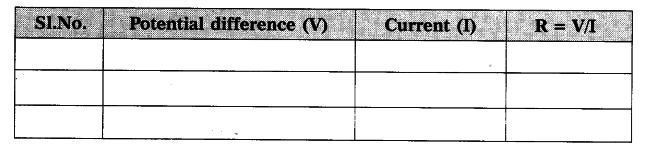# State ohm's law, suggest an experiment to verify it and explain the procedure

Ohm s law:
The current through a conductor element is proportional to the potential difference applied between its ends provided the temperature remains constant.

Aim : To show that the ratio of V/I is a constant for a conductor.
Materials required : 5 dry cells of 1.5 V each, conducting wires, an ammeter, a voltmeter, thin iron spoke of length 10 cm, LED and key.
Procedure :
i) Connect a circuit as shown in the figure.
ii) Solder the conducting wires to the ends of the iron spoke and close the key.
iii) Note the reading of ammeter (current) and voltmeter (potential difference) and tabulate them.iv) Now connect two cells in the circuit and note the respective readings of ammeter and voltmeter in the above table.
v) Repeat the above procedure using three cells, four cells and five cells respectively.
vi) Record the values of potential difference and current© corresponding to each case in the above table.
V
vii) Find V/I for each set of values.

viii) We notice that V/I is constant.
ix) From this we can conclude that potential difference between the ends of the iron spoke is directly proportional to the current passing through it. This is ohm’s law.
V
— = constant
I
V\$\propto\$ i